Math Worksheets Preschool Math 1st Grade Math 2nd Grade Math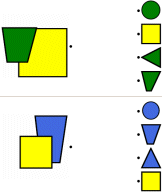Coloring and Matching Shapes

Combined Shapes Review Book
Combined Shapes Review Book (select age level and then print your book)

Coloring Shapes
Not much overlap between two shapes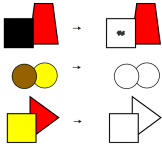Color in one shape - that shape has a color spot      The first problem has only one to color in - rest of problems color both      All problems need to color both shapes

More overlap between two shapes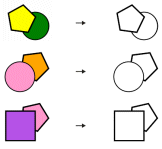Color in one shape - that shape has a color spot      The first problem has only one to color in - rest of problems color both      All problems need to color both shapes

Matching Shapes (match the shapes on the left with the shapes on the right)
Draw a line from the left to the two shapes on the right - right side has ONE wrong shape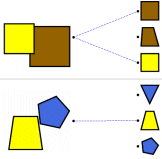First answer is given; rest of problems have one line given      One line given for the first problem      No answers given

Draw a line from the left to the two shapes on the right - right side has TWO wrong shapes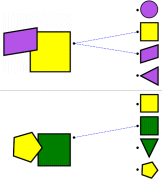First answer is given; rest of problems have one line given      One line given for the first problem      No answers given

More overlap: ONE wrong shape on the right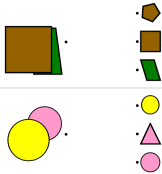First answer is given; rest of problems have one line given      One line given for the first problem      No answers given

More overlap: TWO wrong shapes on the rightFirst answer is given; rest of problems have one line given      One line given for the first problem      No answers given

Have a suggestion or would like to leave feedback?